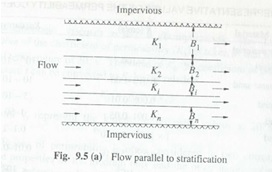Categories

# DARCY’S LAW

Darcy’s law – In 1856 Henry Darcy, a French hydraulic engineer, on the basis of his experimental findings proposed a law relating the velocity of flow in a porous medium. This law know as Darcy’s law can be expressed as

V = Ki

Where V= apparent velocity of discharge =Q/A Q= Discharge

A= Area of seepage medium

K  =  a  coefficient,  called  coefficient  of  permeability    having  the  units  of velocity.

The discharge Q can be expressed as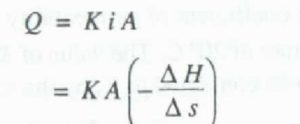Where –  H ( is the drop in the hydraulic  grade line in a length      s of the porous medium.

Darcy’s law is a particular case of the general viscous fluid flow. It has been valid for  laminar flows only. For practical purposes  the limit of the validity of Darcy’s law can be taken as Reynolds number of value unity, i.e.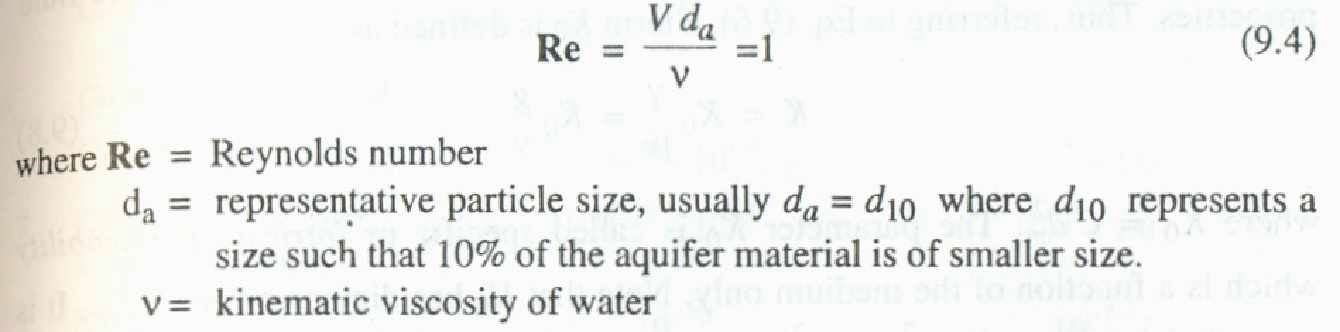Excepting for flow in fissures and caverns, to a large extent groundwater flow in  nature  obeys  Darcy’s  law.  Further,  there  is  no  known  lower  limit  for  the applicability of Darcy’s law.

It may be noted that the apparent velocity V used in Darcy’s law is not the actual velocity of flow through the pores. Owing to irregular pore geometry the actual velocity of flow varies from point to point and the bulk pore velocity (Vs) which represents the actual speed of travel of water in the porous media is expressed as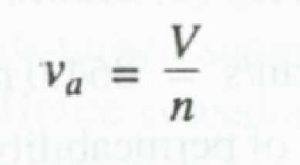where n = porosity. The bulk pore velocity v is the velocity that is obtained by tracking a tracer added to the groundwater.

## Coefficient of Permeability

The  coefficient  of  permeability  also  designated  as  hydraulic  conductivity reflects the combined  effects of the porous medium and fluid properties.  From an analogy of laminar flow through a conduit (Hagen—P o iseuille flow) the coefficient of Permeability can be expressed as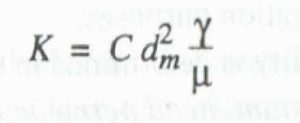The coefficient of permeability is determined in the laboratory by a permeameter . For coarse grained  soils a constant  head permeability  is used. In this the discharge  of water percolating under a constant head difference ( H) through a sample, of porous material of cross. area A and length l is determined. The coefficient of permeability at the temperature of the experiment is found as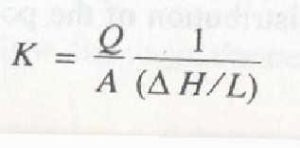For fine grained soils, falling head permeameter is used. It should be noted that laboratory samples are disturbed samples and a permeameter cannot simulate the field conditions exactly. Hence considerable care in the preparation of the samples and in conducting the tests are needed to obtain meaningful results.

Under  field  conditions,  permeability  of  an  aquifer  is  determined  by  conducting pumping tests in a well. One of the many tests available for this purpose consists of pumping out water from a well at a uniform rate till steady state is reached. Knowing the steady state drawdown and the discharge rate, transmissibility can be calculated. Information   about the thickness of the saturation zone leads one to calculate the Permeability. Injection of a tracer, such as a dye and finding its velocity of travel is another way of determining the permeability under field conditions.

Sometimes the aquifers may be stratified, with different permeabilities in each-strata. T kinds of flow situations are possible in such a case.

(i)  When  the  flow  is  parallel  to  the  stratification  as  in  Fig.  9.5  (a)  equivalent

Permeability Ke of the entire aquifer of thickness